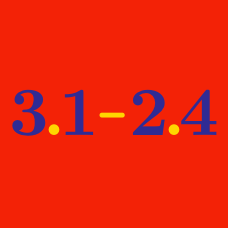Everyday Math

# Decimals: Level 1 Challenges

We see that the fractional part of $\frac{1}{3}$ is 0.333... It repeats after one digit. Let us say that $\frac{1}{3}$ has "period" one. We can also say that $\frac{1}{7} = 0.142857142857142857...$ has "period' six. Which of the following has the longest "period'?

Order these decimals and fractions. Your answer should be the largest one followed by the smallest one.

$\dfrac{3}{4}$

$.83$

$\dfrac{86}{100}$

$.79$

PS. The numbers aren't in order for biggest and smallest

$\large \displaystyle {0. \overline{42}-0.\overline{35}= \, ?}$


Note: $0.\overline{ab}=0.abababab \ldots$

$\large (1.2 \times 1.5) - \dfrac{0.32}{0.8} = \, ?$

Even though the digits of the decimal $\Large \color{#3D99F6}{10.5555555555}\ldots$ repeat forever, it can be written a simple fraction! Which of these fractions is equivalent to this decimal number?

×